# CD Set Delays to Model VI

LabVIEW 2018 Control Design and Simulation Module Help

Edition Date: March 2018

Part Number: 371894J-01

»View Product InfoDownload Help (Windows Only)

Owning Palette: Model Information VIs

Requires: Control Design and Simulation Module

Sets the given delays in the system model. Wire data to the State-Space Model In input to determine the polymorphic instance to use or manually select the instance.

Use the pull-down menu to select an instance of this VI.

 Select an instance CD Set Delays to Model (State-Space)CD Set Delays to Model (Transfer Function)CD Set Delays to Model (Zero-Pole-Gain)

## CD Set Delays to Model (State-Space)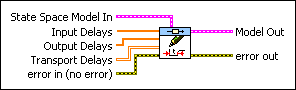State Space Model In contains a mathematical representation of and information about the system for which this VI sets time delays.Input Delays is an array of input delays where the ith element of this array is the time delay in the ith input of the system. If you do not wire an input to Input Delays, the VI uses the input delays from the State-Space Model In input.Output Delays is an array of output delays where the ith element of this array is the time delay in the ith output of the system. If you do not wire an input to Output Delays, the VI uses the output delays from the State-Space Model In input.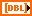Transport Delays is a 2D-array. The ijth element of this array is the time delay between the ith output and jth input pair of the system.error in describes error conditions that occur before this node runs. This input provides standard error in functionality.Model Out is the modified form of the model with its delays set according to the values given in Input Delays, Output Delays, and Transport Delays. To access and modify the data in the model, use the Model Information VIs.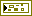error out contains error information. This output provides standard error out functionality.

## CD Set Delays to Model (Transfer Function)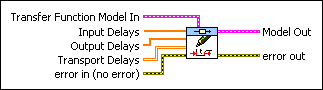Transfer Function Model In contains a mathematical representation of and information about the system for which this VI sets time delays.Input Delays is an array of input delays where the ith element of this array is the time delay in the ith input of the system. If you do not wire an input to Input Delays, the VI uses the input delays from the State-Space Model In input.Output Delays is an array of output delays where the ith element of this array is the time delay in the ith output of the system. If you do not wire an input to Output Delays, the VI uses the output delays from the State-Space Model In input.Transport Delays is a 2D-array. The ijth element of this array is the time delay between the ith output and jth input pair of the system.error in describes error conditions that occur before this node runs. This input provides standard error in functionality.Model Out is the modified form of the model with its delays set according to the values given in Input Delays, Output Delays, and Transport Delays. To access and modify the data in the model, use the Model Information VIs.error out contains error information. This output provides standard error out functionality.

## CD Set Delays to Model (Zero-Pole-Gain)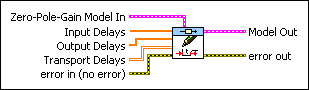Zero-Pole-Gain Model In contains a mathematical representation of and information about the system for which this VI sets time delays.Input Delays is an array of input delays where the ith element of this array is the time delay in the ith input of the system. If you do not wire an input to Input Delays, the VI uses the input delays from the State-Space Model In input.Output Delays is an array of output delays where the ith element of this array is the time delay in the ith output of the system. If you do not wire an input to Output Delays, the VI uses the output delays from the State-Space Model In input.Transport Delays is a 2D-array. The ijth element of this array is the time delay between the ith output and jth input pair of the system.error in describes error conditions that occur before this node runs. This input provides standard error in functionality.Model Out is the modified form of the model with its delays set according to the values given in Input Delays, Output Delays, and Transport Delays. To access and modify the data in the model, use the Model Information VIs.error out contains error information. This output provides standard error out functionality.